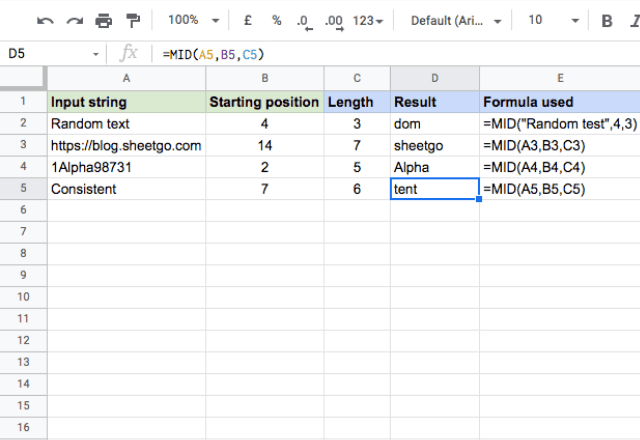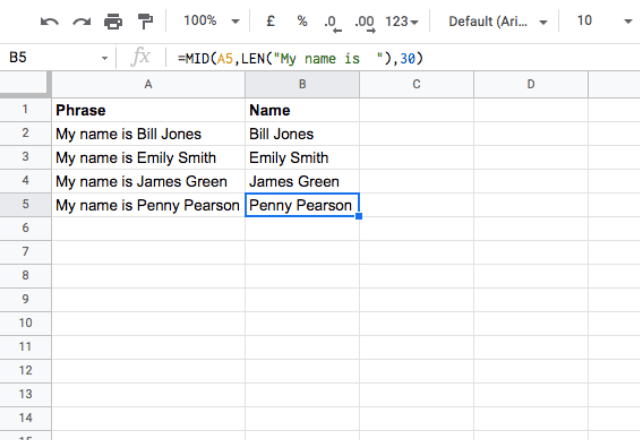# How to use the MID formula in Google SheetsYou can use spreadsheets not only for number crunching but for operating with text-based data as well. Google Sheets has a built-in support system to easily convert, trim and combine text strings. You may already be familiar with formulas like LEFT and RIGHT that help extract substrings from the left and right sides of a string respectively.

What if you need to extract something in between? You can do so using the MID formula in Google Sheets.

### Syntax

=MID(string, starting_at, extract_length)
• string – is the input text that you need to extract a substring from.
• starting_at – is the position from the left of string from which to begin extracting. Notate the first character of the string with position 1.
• extract_length – specifies the number of characters to extract from the position defined by starting_at.

### Usage: MID function in Google Sheets

Nothing beats examples when it comes to understanding the concepts. So, I’ll dive right in to reinforce what you’ve learned from the syntax.

Take a look at the image below.To understand these examples easily, think of the output values as a segment cut out from an input string. In the first example, the formula picked the result “dom” from “Random text” by going to the 4th character and extracting the text for a length of 3 characters. A similar process was repeated in the next two examples.

What if the formula reaches the end of the string before it extracts the extract_length completely? The formula simply returns characters from starting_at to the end of the string. So instead of generating an error, it just stops at the end of the string. I demonstrated this in the final example. The total length of the string “Consistent” is 10. I wanted the formula to start at the 7th position, and extract 6 characters after that. That implies that the formula should go until 12th character, which doesn’t exist. So the formula just returned “tent”, because that is what is available until the end of the input string.

#### Combining MID with the LEN function

It’s very useful to combine text functions together to dynamically extract information. While this is often done with the LEFT and RIGHT functions, you can also use MID to accomplish this.

In the example below, I have names embedded in sentences. I use the MID and LEN functions to extract the names only, with the formula =MID(A2,LEN(“My name is “),30).The LEN function determines the length of the first part of the phrase, then tells the MID function where to start extracting the substring. I set the extract_length to 30 to be sure to get the full length of the name.

That’s it! While MID is a fairly simple function, I hope you can now use it to make your own spreadsheets more efficient.

Editor’s note: This is a revised version of a previous post that has been updated for accuracy and comprehensiveness.